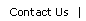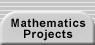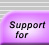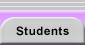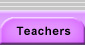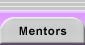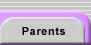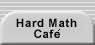# Number Theory

Number theory is the study of the properties of the integers. Its topics include divisibility and primality, irrational numbers, the representation of numbers, Diophantine equations, and much more.

A quick and helpful survey of basic number theory ideas that would be useful for a number of research projects can be dowloaded from A Guide to Arithmetic.

Many interesting number theory discussions can be found at Cut-the-Knot.

An eclectic sample of number theory problems is presented at Number Theory.

Everything you always wanted to know about prime numbers can be found at The Prime Number Site.

For specific topics, see:

Euclid's Algorithm

Fermat's Little Theorem

Chinese Remainder TheoremTranslations of mathematical formulas for web display were created by tex4ht. © Copyright 2003 Education Development Center, Inc. (EDC)